.NET Framework 4.5.2+

# Worksheet.Rows Property

Returns the collection of all rows in a worksheet.

## Declaration

``RowCollection Rows { get; }``

## Property Value

Type Description
RowCollection

The collection of Row objects.

## Remarks

Use the RowCollection object’s members to access, add, hide, group, and remove rows.

### Access Rows

Use the RowCollection.Item property to access an individual row in a worksheet.

#### Obtain the Row at the Specified Index

``````using DevExpress.Spreadsheet;
// ...

// Access the row collection.
RowCollection rows = workbook.Worksheets.Rows;

// Access the first row in the collection.
Row firstRow = rows;
``````

A row index is zero-based. It specifies the row position in the collection.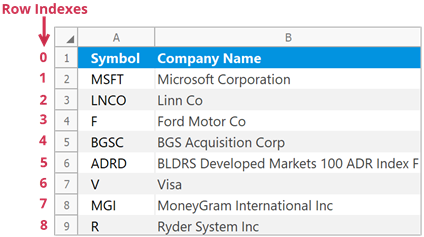#### Obtain the Row with a Given Heading

``````using DevExpress.Spreadsheet;
// ...

// Access the row collection.
RowCollection rows = workbook.Worksheets.Rows;

// Access the first row by its heading.
Row firstRow = rows["1"];
``````

Row headings help users identify each row in a worksheet.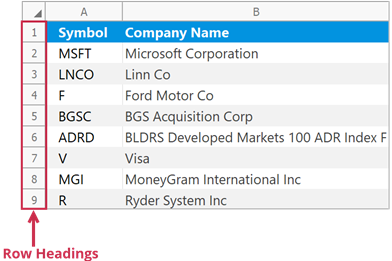### Insert Rows

Use the following methods to insert rows into a worksheet:

Row.Insert
Inserts a row above the current row.
RowCollection.Insert
Inserts rows at the specified position.

Note

The number of rows in a worksheet does not change - 1,048,576. When you add new rows, the rows below are shifted down and an equivalent number of rows at the end of the worksheet is removed.

#### Insert a Single Row

``````// Insert the third row.
worksheet.Rows["3"].Insert();

// Insert the fifth row.
worksheet.Rows.Insert(4);
``````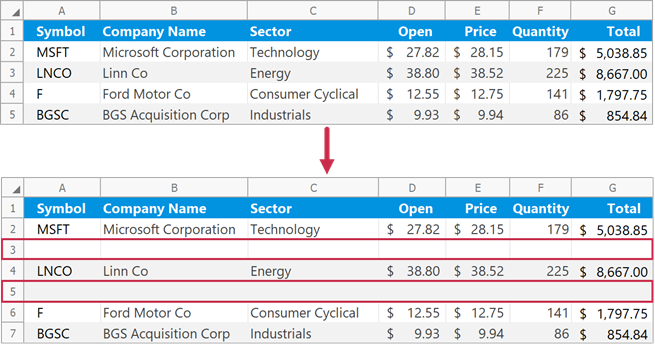#### Insert Multiple Rows

``````// Insert three rows (from row 3 to row 5).
worksheet.Rows.Insert(2, 3);
``````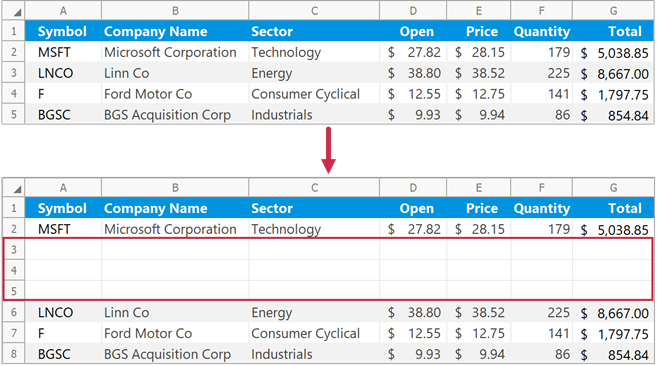#### Insert Rows Above a Specific Cell Range

Use the Worksheet.InsertCells method to insert empty rows above the specified cells. The method inserts the same number of rows as the specified cell range.

``````// Insert two rows above the "B3:E4" cell range.
worksheet.InsertCells(worksheet.Range["B3:E4"], InsertCellsMode.EntireRow);
``````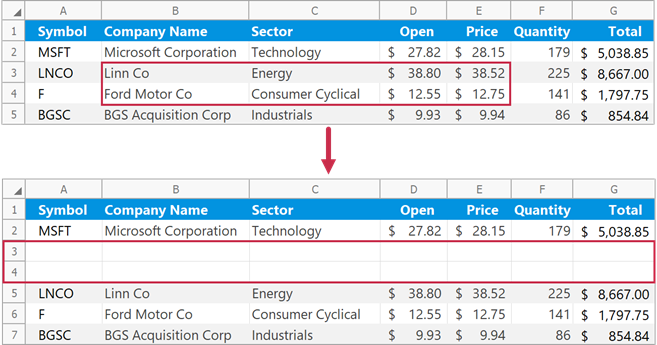#### Format Inserted Rows

Pass a RowFormatMode enumeration member to the RowCollection.Insert method to specify format options for inserted rows.

``````// Insert two rows with the same formatting as the first row.
worksheet.Rows.Insert(1, 2, RowFormatMode.FormatAsPrevious);
``````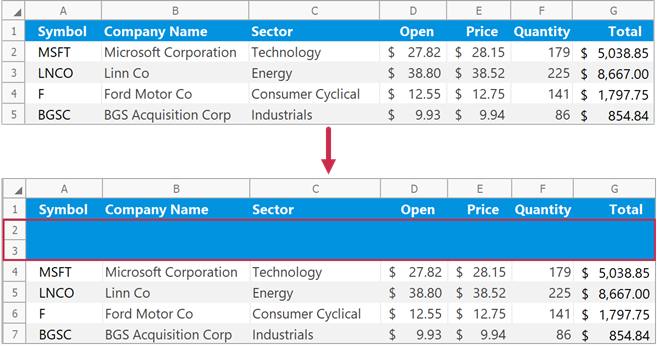### Remove Rows

Use the following methods to delete rows from a worksheet:

Row.Delete
Removes the current row.
RowCollection.Remove
Removes rows at the specified position.

Note

The number of rows in a worksheet does not change - 1,048,576. When you delete rows, the rows below are shifted up and an equivalent number of new rows is added to the end of the worksheet.

#### Remove a Single Row

``````// Delete the second row.
worksheet.Rows.Delete();

// Delete the third row.
worksheet.Rows.Remove(2);
``````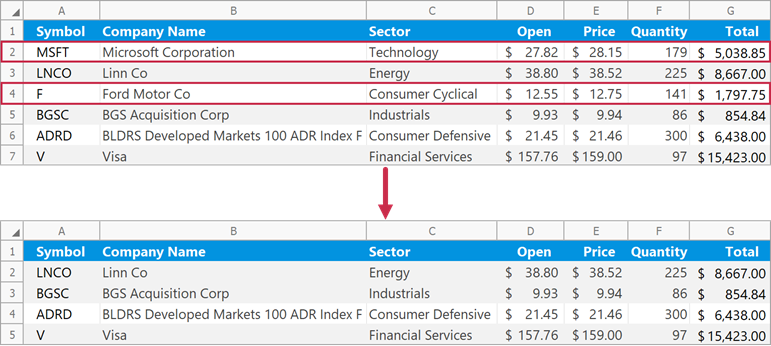#### Remove Multiple Rows

``````// Delete three rows (from row 2 to row 4).
worksheet.Rows.Remove(1, 3);
``````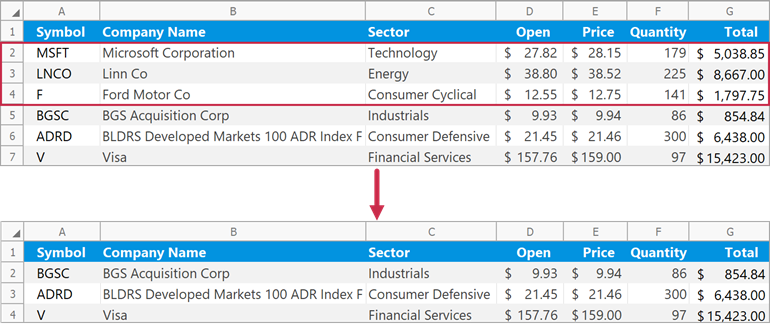#### Remove Rows That Meet a Specific Condition

``````// Specify the condition to remove worksheet rows.
// If a value in the "D" column is greater than 30, remove the corresponding row.
Func<int, bool> rowRemovalCondition = x => worksheet.Cells[x, 3].Value.NumericValue > 30.0;

// Delete rows that meet the specified condition.
// Check rows 2 through 7.
worksheet.Rows.Remove(1, 6, rowRemovalCondition);
``````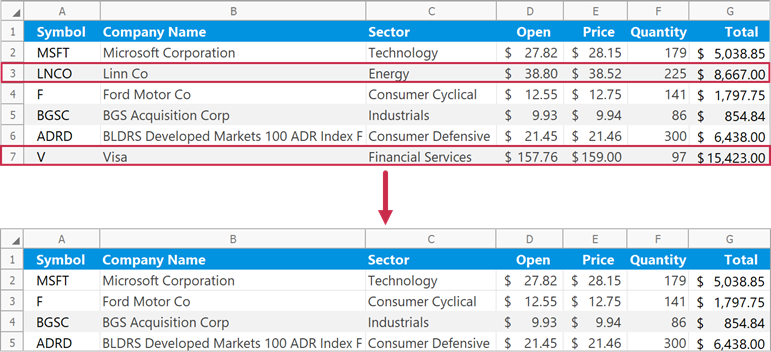#### Remove Rows That Contain Specific Cells

Use the Worksheet.DeleteCells method to delete rows that contain the specified cell range.

``````// Delete a row that contains the "B2" cell.
worksheet.DeleteCells(worksheet.Cells["B2"], DeleteMode.EntireRow);
``````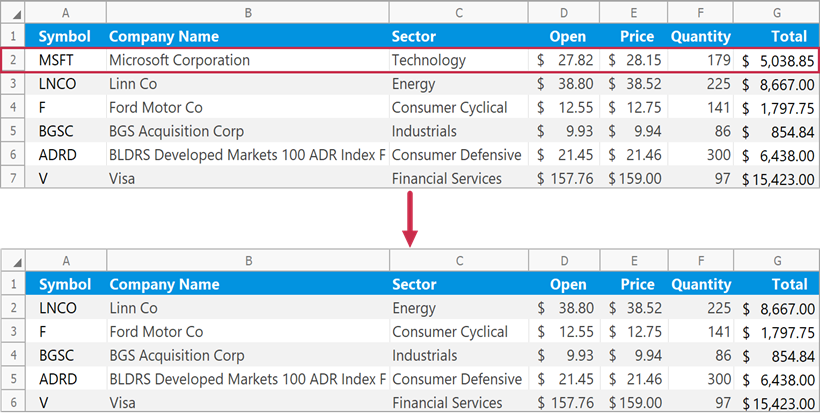### Group and Ungroup Rows

Use the RowCollection.Group method to group rows.

``````// Group rows 3 through 6 and collapse the group.
worksheet.Rows.Group(2, 5, true);

// Group rows 9 through 12 and expand the group.
worksheet.Rows.Group(8, 11, false);

// Group rows 2 through 13 to create the outer group.
worksheet.Rows.Group(1, 12, false);
``````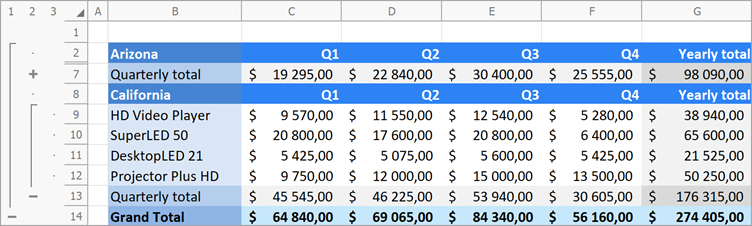Use the RowCollection.UnGroup method to ungroup rows.

``````// Ungroup rows 3 through 6 and display collapsed data.
worksheet.Rows.UnGroup(2, 5, true);

// Ungroup rows 9 through 12.
worksheet.Rows.UnGroup(8, 11, false);

// Remove the outer row group.
worksheet.Rows.UnGroup(1, 12, false);
``````

#### Expand or Collapse Groups

Use the following methods to collapse or expand row groups in a worksheet:

RowCollection.CollapseAllGroups
Collapses all row groups.
RowCollection.CollapseGroups
Collapses row groups starting with the specified group level.
RowCollection.ExpandAllGroups
Expands all row groups.
``````// Collapse all row groups except the first level group.
worksheet.Rows.CollapseGroups(2);
``````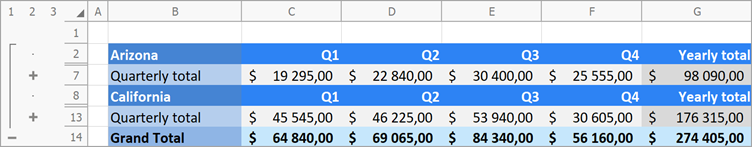### Hide Rows

Use the Row.Visible property or the RowCollection.Hide method to hide rows in a worksheet.

``````// Hide rows 2 through 6.
worksheet.Rows.Hide(1, 5);

// Hide row 8.
worksheet.Rows.Visible = false;
``````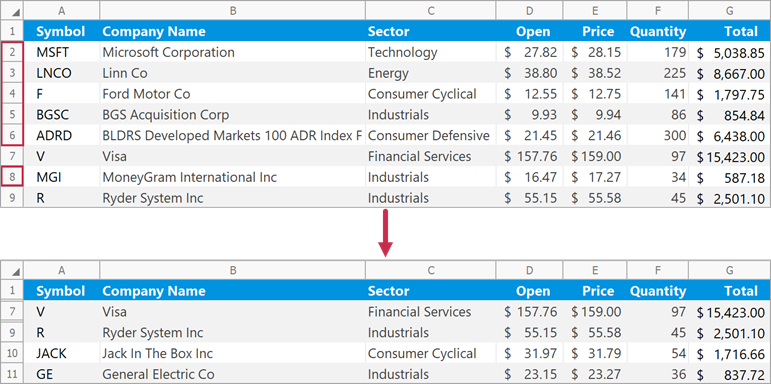Set the Row.Visible property to true to display a row. Use the RowCollection.Unhide method to show multiple hidden rows.

``````// Show rows 2 through 6.
worksheet.Rows.Unhide(1, 5);

// Show row 8.
worksheet.Rows.Visible = true;
``````

The following code snippets (auto-collected from DevExpress Examples) contain references to the Rows property.

Note

The algorithm used to collect these code examples remains a work in progress. Accordingly, the links and snippets below may produce inaccurate results. If you encounter an issue with code examples below, please use the feedback form on this page to report the issue.